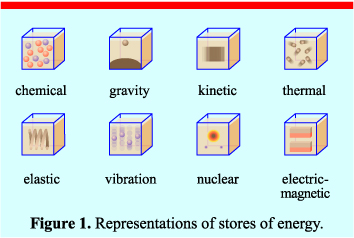# Energy Stores and Systems

## What Is Energy

Defining energy can be very difficult. Simply put it is a property that must be transferred to an object in order to perform work or heat on that object. This means that energy is required for stuff to happen.

Energy is measured in Joules (J). This is the same for all of the different energy stores. Whether it is kinetic or chemical, it is still measured in Joules.

Energy will often be described as part of an ‘energy system’. A system is an object or a group of objects. Therefore when describing the changes within an energy system, you only need to write about one or two objects and the surroundings.

## Energy Stores

There are eight different energy stores which might be mentioned in your GCSE:1. Chemical store of energy, for example batteries and food
2. Gravitational store of energy, for example any object that can fall such as a aeroplane or a ball that has been thrown
3. Kinetic store of energy, for example any object that is moving such as a person running
4. Elastic store of energy, for example any object that has been stretched (extended) or 5. compressed (squashed)
5. Thermal store of energy, for example any object giving off heat such as a radiator
6. Vibrational store of energy, for example any object that is producing sound such as a person’s voice
7. Nuclear store of energy, for example a nuclear power station or a nuclear bomb
8. Magnetic and Electric store of energy, this is for magnetic and electric fields.

## Energy Transfers

Energy can be transferred from one store to another by a number of different ways. These include:

1. Light
2. Sound
3. Heating
4. Work done
5. Electric Current

These are sometimes described as energy pathways. The ones in __bold __you will have to calculate, these will be discussed later in the GCSE revision resources.

## Examples of Energy Transfers

There are some examples for energy transfers for common situations which you need to know for the exam.

1. An object being projected (thrown) upwards

In this example the object starts with kinetic energy. As it travels upwards, the kinetic energy gradually changes into gravitational energy until the highest point. Here, all the kinetic energy has been transferred into gravitational energy.

As the object starts to fall, the energy in the gravitational store transfers into the kinetic store until it hits the ground, where all of the gravitational energy has been transferred into kinetic energy

2. A moving object hitting an obstacle

Here, we have an object full of kinetic energy. Once it hits an obstacle this energy is quickly dissipated and changes into thermal energy and vibrational energy. We know this to be the case since a collision involves thermal energy and the sound produced is from the vibrational store.

3. An object accelerated by a constant force

In this case, the kinetic energy of the object is always increasing as the speed of the object is also increasing. The energy that is transferred into kinetic energy could be a range of examples but the most common one will be chemical. This is because both petrol in an engine and our muscles both start with a chemical store of energy,

## Conservation of Energy

The Conservation of Energy is one of the most important laws in all of Science, not just Physics!

It states that:

“Energy cannot be created or destroyed, only transferred from one store to another”

Remember this!!

Using this law we can say the following:

If 100J of chemical energy from petrol in car is transferred to 80J of kinetic energy, how much energy is wasted?

The answer is 100J - 80J = 20J

This must be the answer since no energy is destroyed. In this example, the 20J of energy is thermal energy which is released to the surroundings.

What is the unit of Energy?